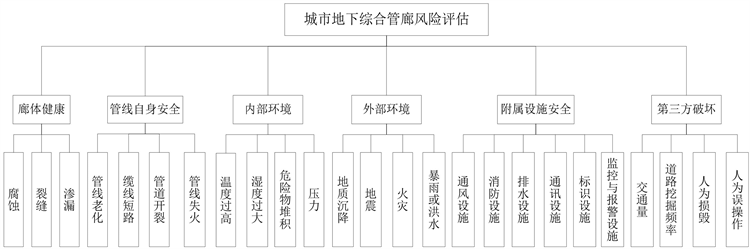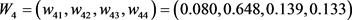﻿ 基于模糊层次分析法的城市地下综合管廊风险评估

# 基于模糊层次分析法的城市地下综合管廊风险评估Risk Assessment of Urban Underground Utility Tunnel Based on Fuzzy Analytic Hierarchy Process

Abstract: As an integrated urban resource transportation system, the underground utility tunnel brings convenience to the live of urban residents, and there are also hidden dangers of security risks that cannot be ignored. There are many factors that affect the safety of urban underground utility tunnel, and these factors have the characteristics of fuzziness and uncertainty. Based on the actual situation and considering the various factors, this paper constructs a risk assessment index system of urban underground utility tunnel, which includes 6 primary indicators and 25 secondary indicators. And the analytic hierarchy process and fuzzy comprehensive evaluation method were combined to evaluate the safety of the utility tunnel. Finally, a case is given to prove the feasibility and effectiveness of the method.

1. 引言

2. 城市地下综合管廊风险评估指标体系的构建Figure 1. The risk assessment index system of urban underground utility tunnel

1) 廊体健康

2) 管线自身安全

3) 内部环境

4) 外部环境

5) 附属设施安全

6) 第三方破坏

3. 模糊层次综合评价法

3.1. 建立数学评价模型

3.2. 采用层次分析法求权重

Step 1：建立递阶层次结构。将与实际问题有关的因素分解成若干层次，最上层为目标层，中间依次为准则层和指标层，最下层为方案或对象层。

Step 2：构造层次模型中的判断矩阵。对准则层中的各个因素使用成对比较法和Satty提出的9点标度法构造出成对判断矩阵，记为 $A$ 。同样对于下一级指标层中隶属于准则层中每个因素的同一层诸因素也使用该方法构造出所有的判断矩阵，记为 ${B}_{i}$ 。两两指标进行比较的9级标度含义表示如表1所示。Table 1. 9-level scale meaning of the judgment matrix

$A=\left[\begin{array}{cccc}{u}_{11}& {u}_{12}& \cdots & {u}_{1n}\\ {u}_{21}& {u}_{22}& \cdots & {u}_{2n}\\ ⋮& ⋮& \ddots & ⋮\\ {u}_{n1}& {u}_{n2}& \cdots & {u}_{nn}\end{array}\right]$

Step 3：计算判断矩阵 $A$ 的最大特征值 ${\lambda }_{\mathrm{max}}$ 以及其相应的特征向量，对特征向量进行归一化处理得到权重向量 $W=\left({w}_{1},{w}_{2},\cdots ,{w}_{n}\right)$ 。同样可以得到指标层二级权重向量 ${W}_{n}=\left({w}_{n1},{w}_{n2},,{w}_{nm}\right)$

Step 4：检验判断矩阵的一致性。计算矩阵的一致性比率 $CI$

$CR=\frac{CI}{RI}=\frac{{\lambda }_{\mathrm{max}}-n}{\left(n-1\right)RI}$ (1)Table 2. The average random consistency indicator RI

3.3. 构建评价矩阵

3.4. 模糊层次综合运算

${M}_{i}={W}_{i}·{R}_{i}=\left({w}_{i1},{w}_{i2},\cdots ,{w}_{im}\right)·\left[\begin{array}{cccc}{r}_{11}& {r}_{12}& \cdots & {r}_{1l}\\ {r}_{21}& {r}_{22}& \cdots & {r}_{2l}\\ ⋮& ⋮& \ddots & ⋮\\ {r}_{m1}& {r}_{m2}& \cdots & {r}_{ml}\end{array}\right]=\left({m}_{i1},{m}_{i2},\cdots ,{m}_{il}\right)$ (2)

$M=\left[\begin{array}{c}{M}_{1}\\ {M}_{2}\\ ⋮\\ {M}_{n}\end{array}\right]=\left[\begin{array}{cccc}{m}_{11}& {m}_{12}& \cdots & {m}_{1l}\\ {m}_{21}& {m}_{22}& \cdots & {m}_{2l}\\ ⋮& ⋮& \ddots & ⋮\\ {m}_{n1}& {m}_{n2}& \cdots & {m}_{nl}\end{array}\right]$

$N=W·M=\left({w}_{1},{w}_{2},\cdots ,{w}_{n}\right)\left[\begin{array}{cccc}{m}_{11}& {m}_{12}& \cdots & {m}_{1l}\\ {m}_{21}& {m}_{22}& \cdots & {m}_{2l}\\ ⋮& ⋮& \ddots & ⋮\\ {m}_{n1}& {m}_{n2}& \cdots & {m}_{nl}\end{array}\right]=\left({n}_{1},{n}_{2},\cdots ,{n}_{l}\right)$ (3)

4. 实例分析

4.1. 建立评价模型

4.2. 计算各级指标权重向量

$A=\left[\begin{array}{cccccc}1& 1& 3& 4& 5& 4\\ 1& 1& 2& 5& 7& 5\\ 1/3& 1/2& 1& 3& 6& 4\\ 1/4& 1/5& 1/3& 1& 3& 1\\ 1/5& 1/7& 1/6& 1/3& 1& 1/4\\ 1/4& 1/5& 1/4& 1& 4& 1\end{array}\right]$

${W}_{1}=\left({w}_{11},{w}_{12},{w}_{13}\right)=\left(0.400,0.400,0.200\right)$

${W}_{2}=\left({w}_{21},{w}_{22},{w}_{23},{w}_{24}\right)=\left(0.196,0.073,0.196,0.535\right)$

${W}_{3}=\left({w}_{31},{w}_{32},{w}_{33},{w}_{34}\right)=\left(0.469,0.137,0.079,0.315\right)$${W}_{5}=\left({w}_{51},{w}_{52},{w}_{53},{w}_{54},{w}_{55},{w}_{56}\right)=\left(0.218,0.267,0.128,0.098,0.116,0.173\right)$

${W}_{6}=\left({w}_{61},{w}_{62},{w}_{63},{w}_{64}\right)=\left(0.307,0.519,0.120,0.054\right)$

4.3. 建立综合评价矩阵Table 3. The risk fuzzy evaluation table of urban underground utility tunnel

4.4. 模糊综合评价

${M}_{1}={W}_{1}·{R}_{1}=\left(0.400,0.400,0.200\right)·\left[\begin{array}{ccccc}0.2& 0.3& 0.2& 0.2& 0.1\\ 0.1& 0.2& 0.3& 0.2& 0.2\\ 0.3& 0.2& 0.2& 0.2& 0.1\end{array}\right]=\left(0.18,0.24,0.24,0.2,0.14\right)$

${M}_{2}=\left(0.1608,0.2608,0.3196,0.1857,0.0731\right)$

${M}_{3}=\left(0.1096,0.2178,0.3178,0.2452,0.1096\right)$

${M}_{4}=\left(0.0352,0.1352,0.3213,0.3515,0.1568\right)$

${M}_{5}=\left(0.1222,0.2263,0.3391,0.2132,0.0992\right)$

${M}_{6}=\left(0.2029,0.2946,0.3158,0.1174,0.0693\right)$

$M=\left[\begin{array}{c}{M}_{1}\\ {M}_{2}\\ {M}_{3}\\ {M}_{4}\\ {M}_{5}\\ {M}_{6}\end{array}\right]=\left[\begin{array}{ccccc}0.1800& 0.2400& 0.2400& 0.2000& 0.1400\\ 0.1608& 0.2608& 0.3196& 0.1857& 0.0371\\ 0.1096& 0.2178& 0.3178& 0.2452& 0.1096\\ 0.0352& 0.1352& 0.3213& 0.3515& 0.1568\\ 0.1222& 0.2263& 0.3391& 0.2132& 0.0992\\ 0.2029& 0.2946& 0.3158& 0.1174& 0.0693\end{array}\right]$

$\begin{array}{l}N=W·M\\ \text{\hspace{0.17em}}\text{\hspace{0.17em}}\text{\hspace{0.17em}}\text{\hspace{0.17em}}=\left(0.312,0.317,0.188,0.073,0.034,0.076\right)·\left[\begin{array}{ccccc}0.1800& 0.2400& 0.2400& 0.2000& 0.1400\\ 0.1608& 0.2608& 0.3196& 0.1857& 0.0371\\ 0.1096& 0.2178& 0.3178& 0.2452& 0.1096\\ 0.0352& 0.1352& 0.3213& 0.3515& 0.1568\\ 0.1222& 0.2263& 0.3391& 0.2132& 0.0992\\ 0.2029& 0.2946& 0.3158& 0.1174& 0.0693\end{array}\right]\\ \text{\hspace{0.17em}}\text{\hspace{0.17em}}\text{\hspace{0.17em}}\text{\hspace{0.17em}}=\left(0.1486,0.2336,0.2834,0.1966,0.0905\right)\end{array}$

5. 结论

 王崇锋, 张古鹏. 我国未来城市化发展水平预测研究(2010-2020) [J]. 东岳论丛, 2009, 30(6): 131-133.

 孙云章. 城市地下管线综合管廊项目建设中的决策支持研究[D]: [硕士学位论文]. 上海: 上海交通大学, 2008.

 王军, 陈欣盛, 李少龙, 等. 城市地下综合管廊建设及运营现状[J]. 土木工程与管理学报, 2018, 35(2): 101-109.

 林俊, 丛北华, 韩新, 等. 基于CFD模拟分析的城市综合管廊火灾特性研究[J]. 灾害学, 2010, 25(b10): 374-374.

 陈雍君, 李宏远, 汪雯娟, 等. 基于贝叶斯网络的综合管廊运维灾害风险分析[J]. 安全与环境学报, 2018, 18(6): 2109-2114.

 刘柯汝. 城市地下综合管廊的施工安全风险评价[J]. 价值工程, 2018(31): 110-111.

 韩利, 梅强, 陆玉梅, 等. AHP–模糊综合评价方法的分析与研究[J]. 中国安全科学学报, 2004, 14(7): 86-89.

 朱嘉. 城市综合管廊安全风险辨识及评价体系研究[D]: [硕士学位论文]. 重庆: 重庆交通大学, 2017.

 邓雪, 李家铭, 曾浩健, 等. 层次分析法权重计算方法分析及其应用研究[J]. 数学的实践与认识, 2012, 42(7): 93-100.

 郭金玉, 张忠彬, 孙庆云. 层次分析法的研究与应用[J]. 中国安全科学学报, 2008, 18(5): 148-153.

Top